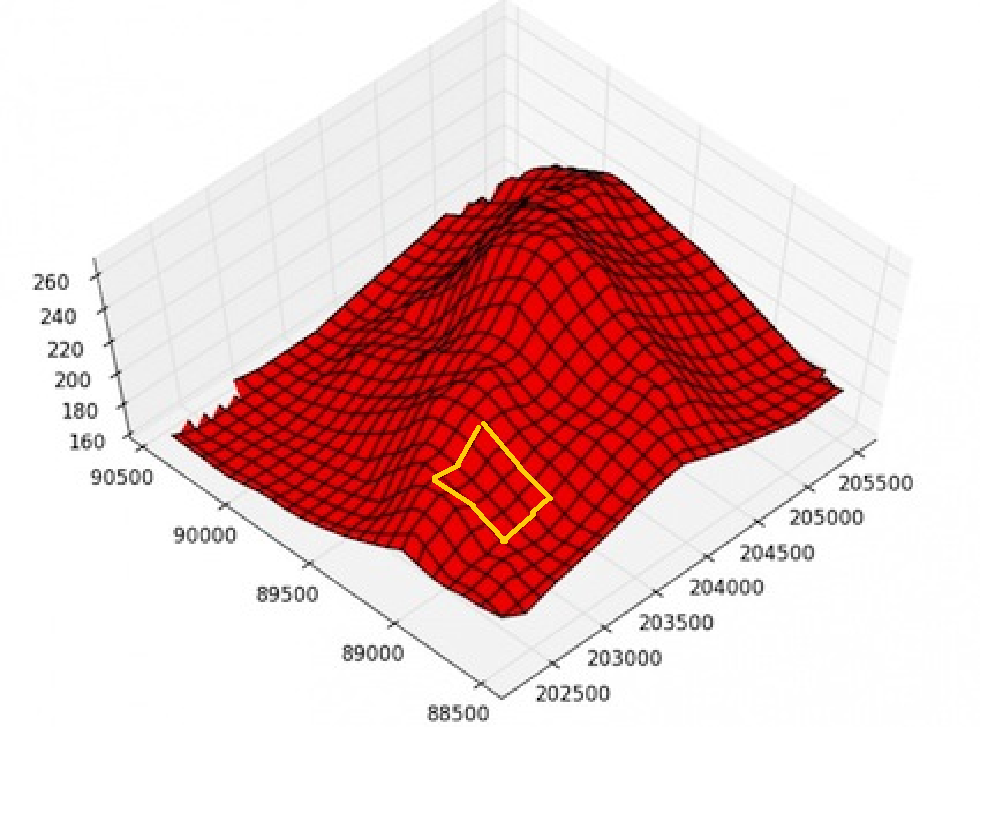# Hi, as a part of an univarsity project i need to do the following with python: - Convert DTM (tiff file) to a matrix of heights - matrix a - Crop a mini DTM from the first one ans convert it to a matrix of heights - matrix b - Display a 3D representati

194
1
10-12-2019 10:06 AMNew Contributor

Hi,

as a part of an univarsity project i need to do the following with python:

- Convert DTM (tiff file) to a matrix of heights - matrix a

- Crop a mini DTM from the first one ans convert it to a matrix of heights - matrix b

- Display a 3D representation of matrix a with matrix b marked on it.

see Image

There is a way to do it with ArcPy? and if so what kind of license do i need?byMVP Esteemed Contributor

you need an 'array' not a matrix... but in any event, you need

``import numpy as npimport arcpyarr = arcpy.RasterToNumPyArray(your_raster_layer_name)‍‍‍‍``

From there you can slice the array to your desired size.

To normalize, you should use numpy functions to do the subtraction etc.

Finally, you can plot the array using matplotlib